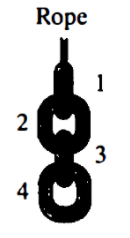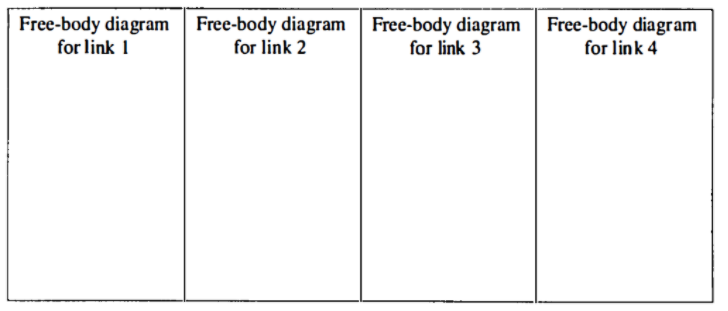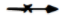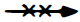# Problem: A chain is suspended by a rope as shown at right. The chain is composed of four identical links and does not move. A. In the spaces below, draw a free-body diagram for each of the four links. Label each of the forces as you were instructed in the tutorial Forces. B. Identify all the Newton's third law (action-reaction) force pairs in your diagrams by placing one or more small "X" symbols through each member of the pair (i.e., mark each member of the first pair as , each member of the second pair as , etc.). C. Rank, from largest to smallest, the magnitudes of all the forces on your diagrams. Explain your reasoning, including how you used Newton's second and third laws.

###### FREE Expert Solution

A.

Free-body diagrams for each of the four links can be presented in the diagram below.

98% (413 ratings)###### Problem Details

A chain is suspended by a rope as shown at right. The chain is composed of four identical links and does not move.A. In the spaces below, draw a free-body diagram for each of the four links. Label each of the forces as you were instructed in the tutorial Forces.B. Identify all the Newton's third law (action-reaction) force pairs in your diagrams by placing one or more small "X" symbols through each member of the pair (i.e., mark each member of the first pair as, each member of the second pair as, etc.).

C. Rank, from largest to smallest, the magnitudes of all the forces on your diagrams. Explain your reasoning, including how you used Newton's second and third laws.